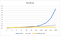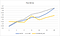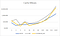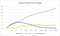# Searching: vector, set and unordered_set

This post is to discuss the performance of finding an element in fixed-size C++11 STL containers with only few elements.

The time complexity to find an element in `std::vector` by linear search is O(N). It is O(log N) for `std::map` and O(1) for `std::unordered_map`. However, the complexity notation ignores constant factors. Different containers have various traversal overheads to find an element. For example, node branching during tree traversals in `std::set` and hashing complexity in `std::unordered_set` are considered constant overheads in complexity. On the other hand, although the complexity of `std::vector`is linear, the memory addresses of elements in `std::vector` are contiguous, which means it is faster to access elements in order.

Therefore, the first idea flashing on me is using `std::vector` to have better performance of searching.

To prove it and for the satisfaction of my curiosity, I did some experiments to test searching speed of the mentioned STL containers. Note that this experiment is not intended to give a specific number of elements for each container with best performance.

The codes could be found at https://gist.github.com/gx578007/836e3ba0069d1570086b0a4c114dca31

# Environment:

• CPU: Intel(R) Core(TM) i5–3337U CPU @ 1.80GHz
• OS: 64 bit Ubuntu 14.04
• Compiler: gcc-4.8.4
• Compiling options: -O3 -std=c++11
• Cache line: 64 bytesInitialization

Each container is initialized with `n` elements in the following ways. This is a one-time job. The finding-element process will repeat ten million times over the initialized containers. The function `rand64()` is implemented to generate a random unsigned 64-bit-integer.

1. std::vector<uint64_t>
`std::vector<uint64_t> c; for (int i=0; i<n; i++)  c.push_back(rand64());`

2. std::set<uint64_t>

`std::set<uint64_t> c;for (int i=0; i<n; i++)  c.insert(rand64());`

3. std::unordered_set<uint64_t>

`std::unordered_set<uint64_t> c;for (int i=0; i<n; i++)   c.insert(rand64());`

Find Elements

For `std::vector`, linear search is applied. Both `std::set` and `std::unordered_set` use `find` to search the target value. The target value will be randomly assigned in each iteration.

1. std::vector<uint64_t>
`// Linear search.for (int i=0; i<1000000; ++i){  uint64_t target = x; // one of existing numbers  for (int k=0; k<c.size(); ++k)    if (c[k] == target)      break;}`

2. std::set<uint64_t>

`for (int i=0; i<1000000; ++i){  uint64_t target = x; // one of existing numbers  c.find(target);}`

3. std::unordered_set<uint64_t>

`for (int i=0; i<1000000; ++i){  uint64_t target = x; // one of existing numbers  c.find(target);}`

# Experiment Results

The runtime of searching to the number of elements is plotted in Fig 1. The y-axis is the runtime in seconds. The x-axis is the number of elements. It can be observed that the performance of all the three containers is a tight race from `n=1` to `n=64`. For `n>=64`, the runtime of `std::vector` linearly increases as `n` increases and `std::set` increases logarithmically. `std::unordered_set` keep a near-constant runtime when `n` increases.

To view the detailed difference between `n=1` and `n=64` clearly, I narrow down the range as shown in Fig 2. What surprises me is that the performance of `std::vector` is not always the best as I thought when `n` is small. Instead, `std::unordered_set` can often have approximately 10% faster than `std::vector` for`n≥4` in my experiments. And the `std::set` is always the worst in that range.Fig. 1. The runtime of ten million searches to the number of elements.Fig. 2. The range from n=1 to n=64 in Fig. 1.

Okay…so what happened ? To dig more about it, I used Linux profiling tool (perf) to monitor cache misses and branch misses during searching, which are shown in Fig. 3 and Fig. 4. respectively.

In Fig. 3, it shows the cache miss number in log scale to the number of elements. For `n≥512`, the cache miss numbers of `std::set` and `std::unordered_set` are much larger than `std::vector`. For `n<=64`, the difference of the cache miss number of these three containers is small. It looks like the caches are well hit for all the containers and I believe this is the main reason why `std::vector` could not outperform other two containers in this range. That is, continuous memory accesses of `std::vector` might not take much advantage when `n` is small in this experiment. However, the fluctuation of cache miss number of `std::set` and `std::unordered_set` could be observed in Fig. 3. The cache miss number of `std::vector` is more stable than the other two. For example, for `n=8`, the cache miss number of `std::unordered_set` suddenly becomes larger than `std::vector` and the corresponding searching speed become worse than `std::vector`.

In Fig. 4, it shows the branch miss percentage to the number of elements. We can say the branch predictions are very precise (>98%) for both`std::vector` and `std::unordered_set`. However, the branch miss percentage of `std::set` is apparently much higher than the other two. Due to the worst performance of `std::set` on cache usage and branch prediction, this might explain why the runtime of `std::set` is the worst.Fig. 3. Number of cache misses in log scale to number of elements.Fig. 4. Branch miss percentage to number of elements. The unit of y-axis is “%”.

In this experiment, the searching result indicates the near best case for each container. With small program size, the code could be well optimized. And most of caches (data caches and instruction caches) and hardware resources are sufficient for the searching operations. Also, the experiment is operated with a single thread so that the penalty of context switches is minimized.

Therefore, in real complex and big programs, the searching performance might get worse. The system-wise factors such as cache misses could have more impacts on searching operations, especially for `std::set` and `std::unordered_set` in terms of cache misses.

In conclusion of searching among few elements, if searching is the only goal or memory usage is a concern, `std::vector` is still my first priority because the advantages of continuous memory accesses, which could reduce the number of cache misses in most cases. And, definitely, if elements will be added or deleted dynamically, `std::unordered_set` and `std::set` will be more proper ones.

Welcome to a place where words matter. On Medium, smart voices and original ideas take center stage - with no ads in sight. Watch
Follow all the topics you care about, and we’ll deliver the best stories for you to your homepage and inbox. Explore
Get unlimited access to the best stories on Medium — and support writers while you’re at it. Just \$5/month. Upgrade